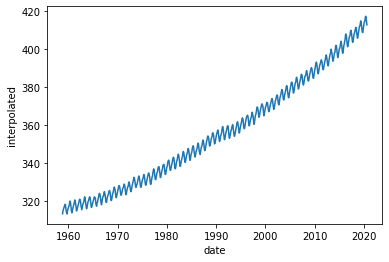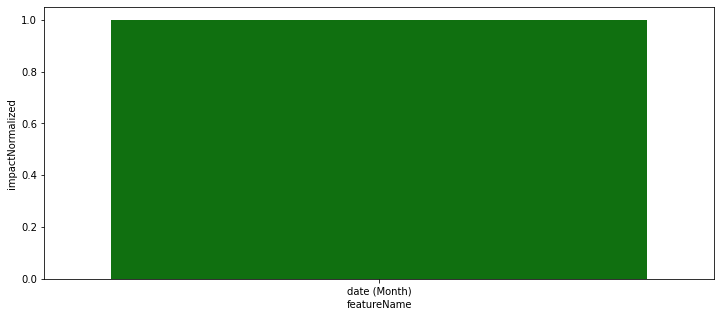Click in-app to access the full platform documentation for your version of DataRobot.

# Predict CO₂ levels with out-of-time validation modeling¶

This notebook demonstrates how to use out-of-time validation (OTV) modeling with DataRobot's Python client to predict monthly CO₂ levels for one of Hawaii's active volcanoes, Mauna Loa. The dataset used in this notebook can be accessed here (select the first dataset listed to emulate results displayed below). For this notebook, the target feature is `interpolated` because `average` has a some missing values that should be skipped.

OTV is a useful modeling method when you know that your data changes in distribution over time. If this is true of your data, random sampling of training and testing datasets would not yield an outcome that would be representative of the model accuracy when it is making predictions in a production environment. Note that OTV can be applied to both classification and regression projects. It partitions your data using the backtesting method, also used in time series modeling.

### Requirements¶

• Python version 3.7.3.
• DataRobot API version 2.21.0.

Small adjustments to the code below may be required depending on the Python version and DataRobot API version used.

Reference documentation for DataRobot's Python client here.

## Import libraries¶

``````import pandas as pd
import datarobot as dr

import seaborn as sns
import matplotlib.pyplot as plt
``````

## Import data¶

``````df = pd.read_csv('<file-path-to-dataset.csv>')
df['day'] = 1 # Displays an arbitrary "day" column to create an accurate "date" feature
``````
year month decimal date average interpolated trend ndays day
0 1958 3 1958.208 315.71 315.71 314.62 -1 1
1 1958 4 1958.292 317.45 317.45 315.29 -1 1
2 1958 5 1958.375 317.50 317.50 314.71 -1 1
3 1958 6 1958.458 -99.99 317.10 314.85 -1 1
4 1958 7 1958.542 315.86 315.86 314.98 -1 1

## Connect to DataRobot¶

Use the snippet below to authenticate and connect to DataRobot. You can read more about different options for connecting to DataRobot from the client.

``````dr.Client(config_path = '<file-path-to-drconfig.yaml')
``````

## Preprocessing¶

Before you begin modeling, you must complete the following steps:

• Create an accurate "date" feature.
• Remove all unnecessary features.
• Create two month lag features.

You can create many more features (such as aggregates on a monthly level or percentages), but for the purposes of OTV, this is not required.

``````df['date'] = pd.to_datetime(df[['year', 'month','day']])
df.drop(['year','month', 'decimal date', 'average', 'ndays','day'],inplace=True, axis=1)

# Create 2 month lag features
for i in range(1,5):
df['lag_{}'.format(i)] = df['interpolated'].shift(i)

df = df.iloc[8:]
``````
interpolated trend date lag_1 lag_2 lag_3 lag_4
8 313.33 315.31 1958-11-01 312.66 313.20 314.93 315.86
9 314.67 315.61 1958-12-01 313.33 312.66 313.20 314.93
10 315.62 315.70 1959-01-01 314.67 313.33 312.66 313.20
11 316.38 315.88 1959-02-01 315.62 314.67 313.33 312.66
12 316.71 315.62 1959-03-01 316.38 315.62 314.67 313.33

## Plot the data¶

By plotting the data (displayed below), you can observe that it follows an upwards trend. Note that randomly partitioning the data for testing purposes would not work and you would not get representative accuracy metrics.

``````sns.lineplot(x='date', y='interpolated',data=df)
``````
````<AxesSubplot:xlabel='date', ylabel='interpolated'>`
```## Define datetime partitioning¶

Use the snippet below to define datetime partitioning for the data. You can also reference a more complete example of Datetime Partitioning.

``````spec = dr.DatetimePartitioningSpecification(datetime_partition_column = 'date',
number_of_backtests = 4,
use_time_series = False)
``````

## Start the project¶

The snippet below passes the `spec` object as an input to the `partitioning_method` variable in the `set_target` method. This starts the project with the designated settings.

``````project = dr.Project.create(df, project_name = 'Predicting CO2 levels for Mauna Loa')

project.set_target('interpolated',partitioning_method = spec, worker_count=-1)
project.wait_for_autopilot()
``````

## Access insights¶

All model insights are available via the API. The example below displays Feature Impact calculated for one of the trained models.

Access more examples and sample code for extracting insights from the DataRobot Community.

``````model = project.get_models()

#Get Feature Impact
feature_impact = model.get_or_request_feature_impact()

#Save feature impact in pandas dataframe
fi_df = pd.DataFrame(feature_impact)
``````
``````fig, ax = plt.subplots(figsize = (12,5))

#Plot feature impact
sns.barplot(x='featureName', y='impactNormalized', data=fi_df[0:5], color='g')
``````
````<AxesSubplot:xlabel='featureName', ylabel='impactNormalized'>`
```Updated June 8, 2022